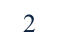Study Guides (390,000)
US (220,000)
KSU (400)
BUS (10)
Final

# BUS 10123 Chapter Notes - Chapter 4: F-Distribution, F-Test, Test StatisticExam

Department
Course Code
BUS 10123
Professor
Eric Von Hendrix
Study Guide
Final

This preview shows page 1. to view the full 5 pages of the document.
1. It can be proved that a t-distribution is just a special case of the more general F-
distribution. The square of a t-distribution with T-k degrees of freedom will be
identical to an F-distribution with (1,T-k) degrees of freedom. But remember that if
we use a 5% size of test, we will look up a 5% value for the F-distribution because the
test is 2-sided even though we only look in one tail of the distribution. We look up a
2.5% value for the t-distribution since the test is 2-tailed.
Examples at the 5% level from tables
T-k F critical value t critical value
20 4.35 2.09
40 4.08 2.02
60 4.00 2.00
120 3.92 1.98
2. (a) H0 :
3 = 2
We could use an F- or a t- test for this one since it is a single hypothesis involving
only one coefficient. We would probably in practice use a t-test since it is
computationally simpler and we only have to estimate one regression. There is one
restriction.
(b) H0 :
3 +
4 = 1
Since this involves more than one coefficient, we should use an F-test. There is one
restriction.
(c) H0 :
3 +
4 = 1 and
5 = 1
Since we are testing more than one hypothesis simultaneously, we would use an F-
test. There are 2 restrictions.
(d) H0 :
2 =0 and
3 = 0 and
4 = 0 and
5 = 0
As for (c), we are testing multiple hypotheses so we cannot use a t-test. We have 4
restrictions.
(e) H0 :
2
3 = 1
Although there is only one restriction, it is a multiplicative restriction. We therefore
cannot use a t-test or an F-test to test it. In fact we cannot test it at all using the
methodology that has been examined in this chapter.
3. THE regression F-statistic would be given by the test statistic associated with
hypothesis iv) above. We are always interested in testing this hypothesis since it
tests whether all of the coefficients in the regression (except the constant) are jointly
insignificant. If they are then we have a completely useless regression, where none
of the variables that we have said influence y actually do. So we would need to go
back to the drawing board!

Unlock to view full version

Only page 1 are available for preview. Some parts have been intentionally blurred.Introductory Econometrics for Finance by Chris Brooks
2
The alternative hypothesis is:
H1 :
2 0 or
3 0 or
4 0 or
5 0
Note the form of the alternative hypothesis: “or” indicates that only one of the
components of the null hypothesis would have to be rejected for us to reject the null
hypothesis as a whole.
4. The restricted residual sum of squares will always be at least as big as the
unrestricted residual sum of squares i.e.
To see this, think about what we were doing when we determined what the
regression parameters should be: we chose the values that minimised the residual
sum of squares. We said that OLS would provide the “best” parameter values given
the actual sample data. Now when we impose some restrictions on the model, so
that they cannot all be freely determined, then the model should not fit as well as it
did before. Hence the residual sum of squares must be higher once we have imposed
the restrictions; otherwise, the parameter values that OLS chose originally without
the restrictions could not be the best.
In the extreme case (very unlikely in practice), the two sets of residual sum of
squares could be identical if the restrictions were already present in the data, so that
imposing them on the model would yield no penalty in terms of loss of fit.
5. The null hypothesis is: H0 :
3 +
4 = 1 and
5 = 1
The first step is to impose this on the regression model:
yt =
1 +
2x2t +
3x3t +
4x4t +
5x5t + ut subject to
3 +
4 = 1 and
5 = 1.
We can rewrite the first part of the restriction as
4 = 1 -
3
Then rewrite the regression with the restriction imposed
yt =
1 +
2x2t +
3x3t + (1-
3)x4t + x5t + ut
which can be re-written
yt =
1 +
2x2t +
3x3t + x4t -
3x4t + x5t + ut
and rearranging
(yt x4t x5t ) =
1 +
2x2t +
3x3t -
3x4t + ut
(yt x4t x5t) =
1 +
2x2t +
3(x3t x4t)+ ut
Now create two new variables, call them Pt and Qt:
pt = (yt - x3t - x4t)
qt = (x2t -x3t)
We can then run the linear regression:
pt =
1 +
2x2t +
3qt+ ut ,